Obtain the data you need to make the most informed decisions by accessing our extensive portfolio of information, analytics, and expertise. Sign in to the product or service center of your choice.

# Two questions and three equations on distance of investigation

24 August 2017 Louis Mattar Hamed Tabatabaie

This is a collaborative article by Hamed Tabatabaie and Louis Mattar.

The distance of investigation concept is often used to answer two different types of questions:

1. How far have we investigated into the reservoir? (At what distance in the reservoir has the pressure been disturbed minimally?)
2. What is the distance to the boundary?

Traditionally, these two questions have been answered using the same equation, namely, the radius of investigation equation. In this equation, t is in hours, and is derived from tDe = 0.25 :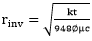This approach has worked well for many years. However, with the appearance of unconventional reservoir systems, which are dominated by linear flow, other distance of investigation equations are being used, and to further confuse the issue, the distance of investigation equations for constant flow rate and constant pressure conditions are different.

There are essentially three equations for distance of investigation in common use:

1. Radial flow (both constant rate and constant pressure): tDe=0.25
2. Linear Flow – constant pressure: tDe=0.25
3. Linear Flow – Constant rate: tDe=0.5

Our investigation into the two Questions, and the three Equations, has resulted in some clarifications, and some unexpected results. These are published in (Tabatabaie et al, 2017) and summarized in this article. The main findings of our research are:

1. The two questions represent two different concepts and should NOT be answered using the same equation.
• Q1 (How far have we investigated) needs its own set of equations.
• Q2 (Distance to boundary) is properly answered using what have been traditionally called “distance of investigation equations”, namely Eq1., Eq.2 and Eq.3.
2. When a pressure disturbance is initiated at the well, there are two issues that are relevant:
1. When does the effect of the disturbance reach a specified location in the reservoir? This is called the Time of Arrival.
2. When can the effect of a boundary be detected at the flowing well? This is called the Time of Detection.
1. At the Time of Arrival, the depletion at the boundary is minimal (<5%)
2. At the Time of Detection, the depletion at the boundary is minimal (<5%) for radial flow, but is substantial (30-40%) for linear flow.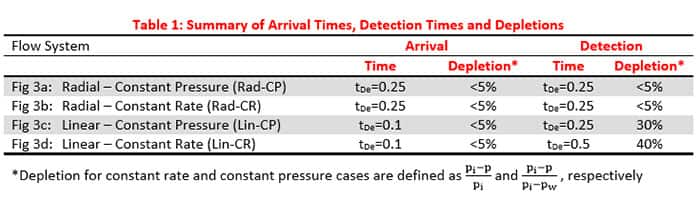Figure 1: Summary of Arrival Times, Detection Times and Depletions

1. Time of Detection should be used for determining distance to a boundary
2. Time of Arrival should be used for determining how far the disturbance has travelled into the reservoir.
3. For radial flow, there is little difference between Time of Arrival and Time of Detection, and that is why historically, a single equation has successfully answered both Q1 and Q2; see Figures 4a and 4b.
4. However for linear flow, the Time of Detection is 2.5-5 times the Time of Arrival !!!; see Figures 4c and 4d.
5. For radial flow the speed of travel of a disturbance is the same for constant rate and for constant pressure – Figure 1a.
6. However for linear flow, the constant pressure pulse appears to travel a little faster than the constant rate pulse – Figures1b and 1c.
7. The “speed of propagation” of a particular depletion depends not only on the hydraulic diffusivity of the medium, but also on the magnitude of the depletion of interest.
8. In summary, the traditional phrase “distance of investigation” being used to represent two different concepts is confusing. To avoid confusion, the phrase “distance of investigation” should be replaced by two different terms, namely:
• distance of influence”: this corresponds to the time of arrival and represents the distance in the reservoir that has been disturbed.
• distance to boundary”: this corresponds to “time of detection” and gives correctly the distance to a boundary (no-flow).

Time of Arrival: The time at which an observer located at a point inside the reservoir detects a measurable pressure difference, typically a pressure difference of <5%.

• The way to determine Time of Arrival is to calculate the pressure profile in the reservoir and note when the pressure at the point of interest declines visibly. See Figures 1a, 1b and 1c.

Time of Detection: The time at which an observer located at the flowing well detects a deviation from infinite acting behavior (senses the boundary). For radial flow, at the time of detection, depletion at the boundary is <5%. However, for linear flow, at the time of detection, depletion at the boundary is 30-40 %!!

• The way to determine Time of Detection is to plot the flowing pressure at the well, and note when it deviates from infinite-acting behavior, see Figures 2a, 2b, 2c and 2d.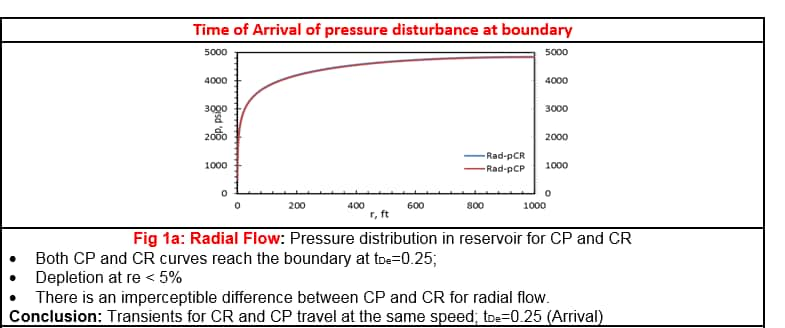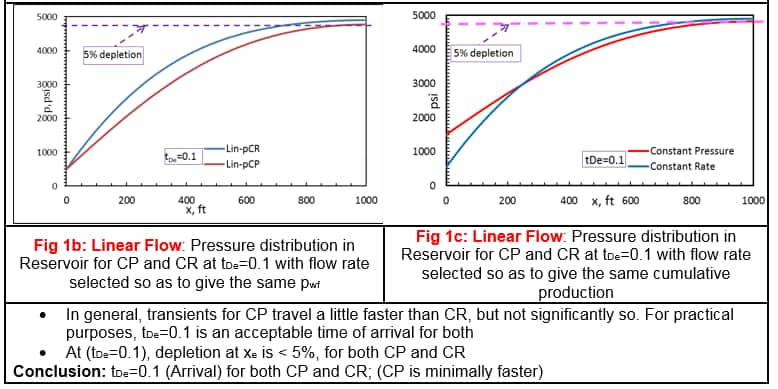Figure 1b and 1c: Linear flow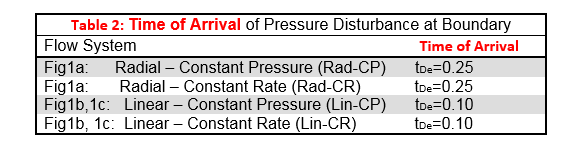Figure 2: Time of arrival of pressure disturbance at boundary

Discussion of Time of Arrival:

Louis Mattar is a Principal Reservoir Advisor at IHS Markit.
Hamed Tabatabaie is a Product Management Principal at IHS Markit.

S. Hamed Tabatabaie is a Product Management Principal and has been involved in reservoir – simulation studies, reserve estimation and production data analysis of conventional and unconventional reservoirs. Hamed’s primary areas of interest include mathematical modeling and production data analysis to optimize hydrocarbon recovery. He earned his BSc and MSc degrees from the Petroleum University of Technology, Iran, and MEng and PhD degrees from the University of Calgary, all in petroleum engineering.

Posted 24 August 2017